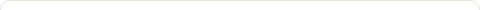# Java Program to Convert Binary to Decimal

Posted on: March 16, 2016

Objectives :

• Binary to Decimal Conversion
• Converting Binary to Decimal
• Write a program to convert binary to decimal
• Write a program to convert binary number to decimal format
• Write a program to convert binary to decimal using Scanner class
• Write a program to convert binary to decimal, take input using Scanner class

Java Program :

```
import java.util.Scanner;

public class BinaryToDecimal {

public static void main(String[] args) {
Scanner scanner = new Scanner(System.in);
System.out.print(&quot;Enter binary number: &quot;);
String binary = scanner.nextLine();
int decimal = binaryToDecimal(binary);
System.out.println(&quot;Decimal equivalent of &quot;+ binary +&quot; is &quot;+ decimal);
}

private static int binaryToDecimal(String binary) {
final int base = 2;
int decimal = 0;
for (int i = 0; i &lt; binary.length(); i++) {
if (binary.charAt(i) == '0') {
decimal += 0 * Math.pow(base, binary.length() - i - 1);
} else if (binary.charAt(i) == '1') {
decimal += 1 * Math.pow(base, binary.length() - i - 1);
} else {
System.out.println(&quot;Invalid Binary Number&quot;);
System.out.println(&quot;Binary Number Contains only 0's or 1's&quot;);
System.exit(0);
}
}
return decimal;
}
}

```

Output :
Enter binary number: 1110
Decimal equivalent of 1110 is 14

### 1 Response to "Java Program to Convert Binary to Decimal"Nice example for binary to decimal conversion in java .
thanks for this post.

Like